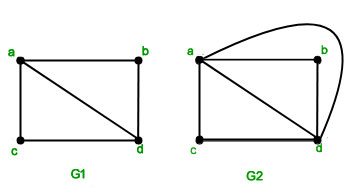Related Articles
GATE | GATE-CS-2005 | Question 10
• Difficulty Level : Medium
• Last Updated : 14 Feb, 2018

Let G be a simple connected planar graph with 13 vertices and 19 edges. Then, the number of faces in the planar embedding of the graph is
(A) 6
(B) 8
(C) 9
(D) 13

Answer: (B)

Explanation:
An undirected graph is called a planar graph if it can be drawn on a paper without having two edges cross and such a drawing is called Planar Embedding. We say that a graph can be embedded in the plane, if it planar. A planar graph divides the plane into regions (bounded by the edges), called faces. Graph K4 is palanar graph, because it has a planar embedding as shown in

figure below.Euler’s Formula : For any polyhedron that doesn’t intersect itself (Connected Planar Graph),the

• Number of Faces(F)
• plus the Number of Vertices (corner points) (V)
• minus the Number of Edges(E)
, always equals 2. This can be written: F + V − E = 2.

Solution :

Here as given, F=?,V=13 and E=19
-> F+13-19=2
-> F=8

So Answer is (B).

This solution is contributed by Nirmal Bharadwaj

We can apply Euler’s Formula of planar graphs. The formula is v − e + f = 2.

Quiz of this QuestionMy Personal Notes arrow_drop_up